Home > CCA2 > Chapter 9 > Lesson 9.3.3 > Problem9-107

9-107.
1. Simplify each expression. Homework Help ✎

1.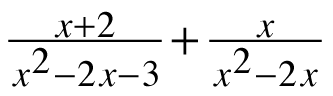2.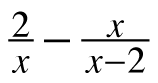3.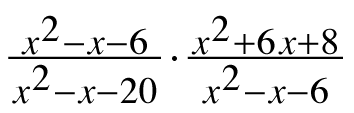4.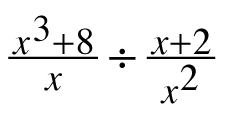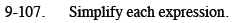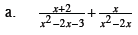Review the Math Notes box in Lesson 3.2.5.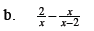$\frac{-x^2+2x-4}{x(x-2)}$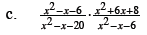Review the Math Notes box in Lesson 3.2.4.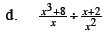Review the Math Notes box in Lesson 8.3.3 for a reminder of how to factor the sum of cubes.